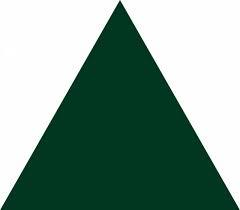# Circumference 4421

The area of an equilateral triangle is 81 times three below the square root of cm2. Calculate its circumference.

x =  54 cm

### Step-by-step explanation:Did you find an error or inaccuracy? Feel free to write us. Thank you!

Tips for related online calculators
The Pythagorean theorem is the base for the right triangle calculator.